Kiekis: 0

Iš viso: 0,00

0

# Solids of revolution

### Solids of revolution

Rotating a geometric shape around a line within its geometric plane as an axis results in a solid of revolution.

Matematika

Raktiniai žodžiai

Kieta revoliucija, nupjautinis kūgis, Kūgis, Cilindras, Sfera, lygiašonis trikampis, Stačiakampis, Vienodo trapecijos, Apskritimas, sudaromoji, Spindulys, šoninis paviršius, bazinis apskritimas, Viršutinis ratas, Kietoji geometrija, Matematika

Susiję elementai

### Vaizdai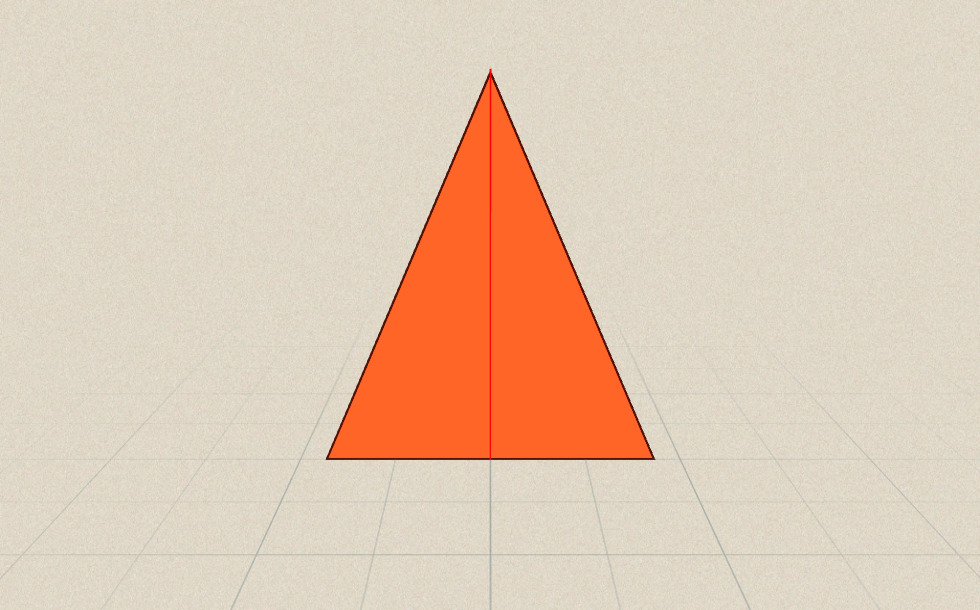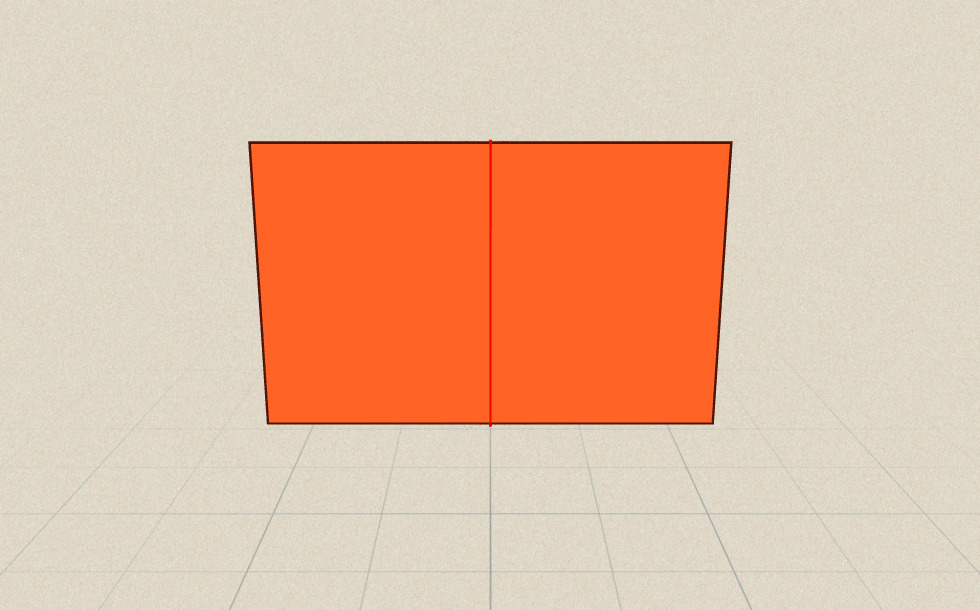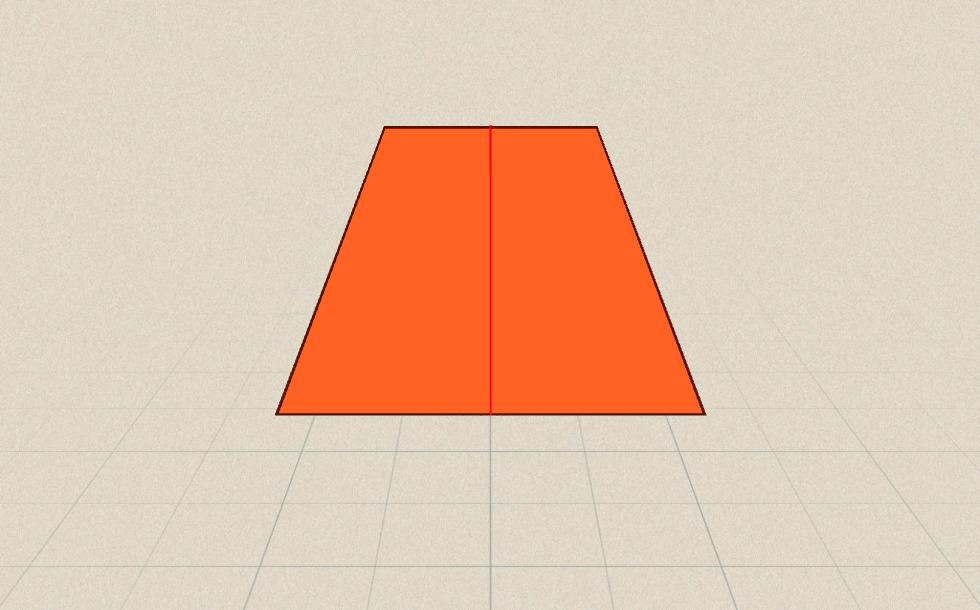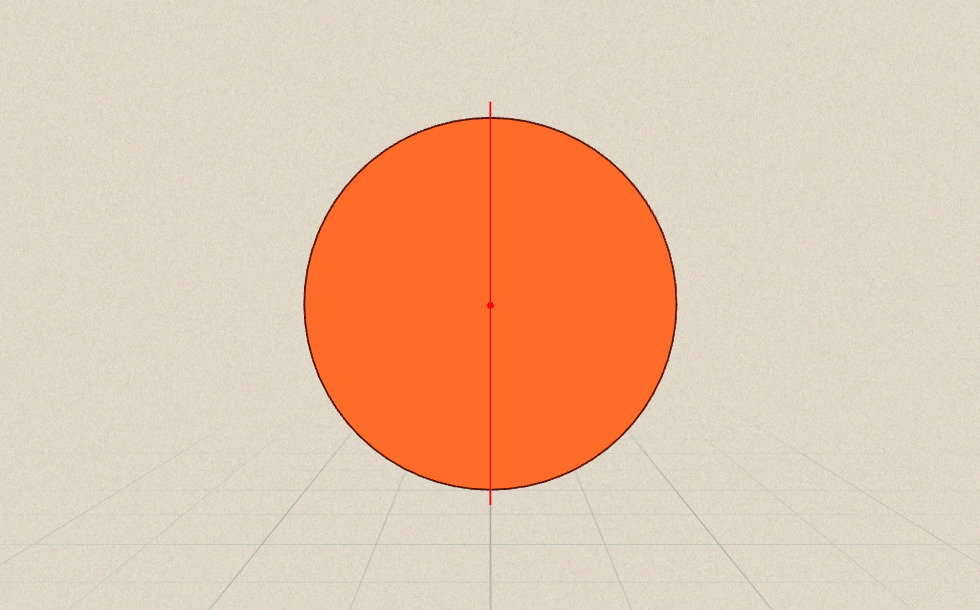### Susiję elementai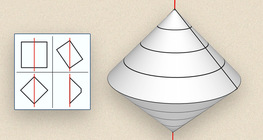#### Solids of revolution (exercise)

An exercise about the generation of solids of revolution.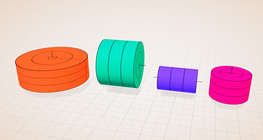#### Solids of revolution (rectangle)

Rotating a rectangle around its axes of symmetry or around its sides results in solids of revolution.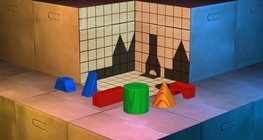This exciting and colourful game is designed to develop spatial perception. You can check your solutions using isometric views.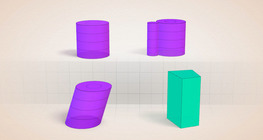#### Cylindrical solids

This animation demonstrates various types of cylindrical solids as well as their lateral surfaces.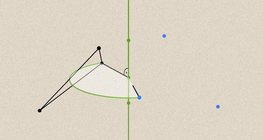#### Geometric transformations – rotation

This animation demonstrates geometric rotation, a type of geometric transformation both in plane and space.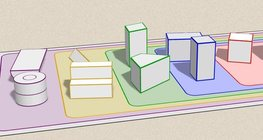#### Grouping of solids

This animation demonstrates various groups of solids through examples.#### Grouping of solids 1

This animation demonstrates various groups of solids through examples.#### Grouping of solids 2

This animation demonstrates various groups of solids through examples.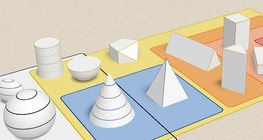#### Grouping of solids 3

This animation demonstrates various groups of solids through examples.#### Grouping of solids 4

This animation demonstrates various groups of solids through examples.#### Non-orientable surfaces

The Möbius strip and the Klein bottle are special two-dimensional surfaces with only one side.#### Perimeter, area, surface area and volume

This animation presents the formulas to calculate the perimeter and area of shapes as well as the surface area and volume of solids.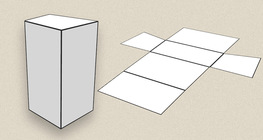#### Prisms

This animation demonstrates several types of prisms, from general to regular.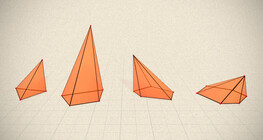#### Regular square pyramid

A regular square pyramid is a right pyramid with a square base and four triangular faces.#### Sphere

A sphere is the set of points which are all within the same distance from a given point in space.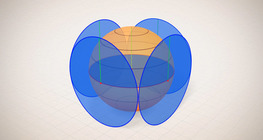#### Surface area of spheres (demonstration)

The surface of a sphere consists of the set of points which are all at the same distance from a given point in space.#### Volume of spheres (Cavalieri´s principle)

Calculating the volume of a sphere is possible using an appropriate cylinder and cone.#### Volume of spheres (demonstration)

The sum of the volume of the ´tetrahedrons´ gives an approximation of the volume of the sphere.#### Conic sections

The conic section is a plane curve that is created when a right circular cone is intersected by a plane.